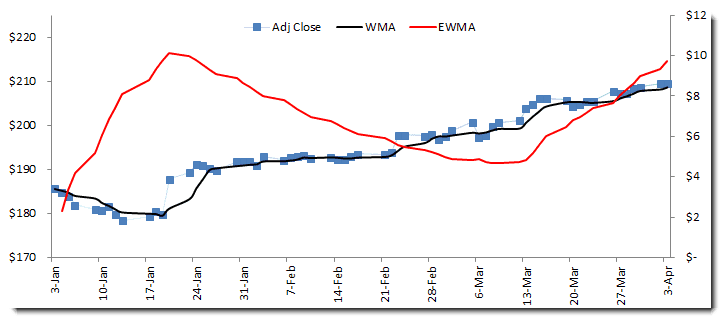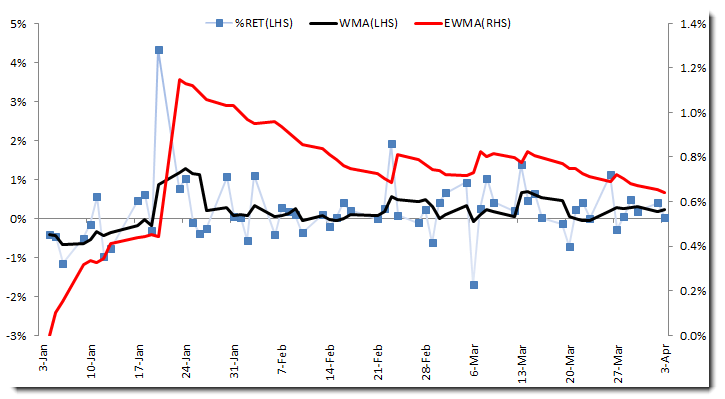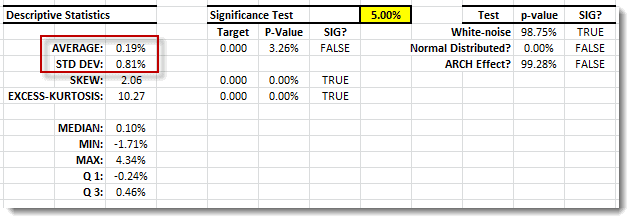# Stationarity

In this issue, the second tutorial in our data preparation series, we will touch on the second most important assumption in time series analysis: Stationarity, or the assumption that a time series sample is drawn from a stationary process.

We’ll start by defining the stationary process and stating the minimum stationery requirements for our time series analysis. Then we demonstrate how to examine sample data, draw a few observations, and highlight the intuitions behind them.

## Background

In a mathematical sense, a stationary process is a stochastic process whose joint probability distribution does not change when shifted in time or space. Consequently, parameters such as the mean and variance, if they exist, also do not change as a result of a shift in time or position. This is often referred to as the strict form of a stationary process.

Let $\left \{X_t \right\}$ be a stochastic process, where $F_x (x_{t_1},x_{t_2},...,x_{t_n})$ is the density (mass) distribution function of the joint distribution of $\left \{ X_t \right \}$. Then $\left \{X_t \right \}$ is said to be stationary if, for all values of shift ($\tau$) and all values of $\left \{t_1,t_2,...,t_N \right \}$,

$$F_x(x_{{t_1}+\tau},x_{{t_1}+\tau},...,x_{{t_N}+\tau})=F_x (x_{t_1},x_{t_2},...,x_{t_n})$$

The $F_x (.)$ function is not affected by a shift across time.

A simplified example would be a Gaussian white-noise process, where each observation is identically distributed and independent from all observations in a given sample. Consequently, the joint probability distribution of the sample data is expressed as follows:

$$F_x (x_{t_1},x_{t_2},...,x_{t_n})=F_x(x_{t_1})\times F_x(x_{t_2})\times...\times F_x(x_{t_N})$$

Furthermore,

$$F_x(x_{t+\tau})=F_x(x_t)$$

So,

$$F_x (x_{t_1},x_{t_2},...,x_{t_n})=F_x(x_{{t_1}+\tau},x_{{t_1}+\tau},...,x_{{t_N}+\tau})$$

The stationary process assumption is very strict and is very difficult to check for outside of a few trivial cases (e.g. white noise). For practical time series analysis, a “weak-sense” stationary process (WSS) is adequate.

## Weak-Sense Stationary (WSS)

A weaker form of a stationary process is called weak-sense stationary (WSS) or covariance stationery. The WSS requires that only the 1st (mean) and second (covariance) moments don’t vary with respect to time.

$$E\left [ x_t \right ]=E\left [ x_{t+\tau} \right ]=\mu$$ $$E\left [ x_t \times x_{t+\tau} \right ]=E\left [x_t \times x_{t-\tau} \right ]=m_x(\tau)$$

The WSS is also referred to as a first-order stationary process. Furthermore, the WSS definition leads to the following conclusions:

1. That the auto-covariance $(\gamma)$ and auto-correlation functions $(\rho)$ are only dependent on $\tau$ (shift over time)
2. The auto covariance $(\gamma)$ and auto-correlation functions $(\rho)$ are dependent on the absolute value of the shift $(\tau)$:

$$\gamma_\tau=\gamma_{-\tau}$$

Note:

For time series analysis; we shall only concern ourselves with the WSS form of a stationary process.

## Checking for a stationary assumption

Let’s assume we have a time series data sample; how do we examine it for stationarity?

1. Visual Method

Before we delve into statistical tests for stationarity, let’s demonstrate in plain words how to examine for stationarity using a time series plot. Keep in mind that we are looking for a relatively stable mean and variance over time. My preferred method is to plot the sample data, moving average, and exponential weighted volatility on the same graph.

• The (weighted) moving average (WMA) is a proxy for the process’s marginal mean.
• The exponential-weighted volatility (EWMA) is a proxy for the process’s marginal standard deviation.

Examine the stability of the mean and variance over time.

Example

Let’s look at the IBM stock daily closing prices process between January 2, 2012, and today (April 3rd, 2012):The graph above shows a trending sample mean but rather stable volatility. As a result, the stationary assumption does not hold for the closing prices process.

Note:

The EWMA function assumes that the process mean is zero(0); however, this is not the case for the closing prices process, so we need to de-mean the series with TSSUB before passing it to EWMA.

Example

Let’s look at the daily log-returns of IBM stock:The daily log returns exhibit a stable mean over time, and the volatility is somewhat bound between 0.6 – 1.2% per trading day.

Note:

We typically ignore the first few EWMA values because the number of observations used to calculate those values is very limited, leading to inaccurate results.The sample data mean is not significantly different than zero, and the volatility (standard deviation) is around 0.8%, which is the centerline for EWMA in our sample (excluding values at the beginning of the sample).

In sum, the IBM stock daily log returns data sample looks stationary.

2. Statistical Test

In practice, the common reason for non-stationarity in sample data is the presence of trend and integration (i.e. unit-root) between the observations themselves.

A number of statistical tests can be utilized to examine the stationary assumptions by decomposing the process into three elements: a deterministic trend, a random walk (unit-root), and a stationary error. The following tests are commonly used to establish stationary assumptions:

1. Trend stationery - Kwiatkowski–Phillips–Schmidt–Shin (KPSS)
2. Unit-root Test or random walk test – Augmented Dickey-Fuller (ADF)

## The stationary assumption is not holding; what can I do?

If a stationary assumption fails to hold, the solution is quite simple: transform the data into a stationary process.

How exactly do we go about making that kind of transformation? Earlier, we mentioned that the presence of trend and/or unit-root (integration) in the time series commonly leads to non-stationarity. Using the statistical test, we can check for the presence of trends and/or unit roots. Next, we apply various techniques including de-trending, seasonal adjustment, and differencing, in order to yield a stationary process.

In financial time series, unit root (random walk) is often found in the raw time series, while the trend may be found in macroeconomic data. An analyst’s experience and familiarity with the type of time series is critical in picking/applying the appropriate transformation techniques.

In the IBM stock closing prices time series, the data showed random-walk behavior. We could also easily compute the ACF functions, and we demonstrated an ACF for lag one with a value as high as 100%. To remove the random walk, we took the first difference and ended up with a stationary process.

IMPORTANT:

We assume that the underlying process has not undergone any structural changes (i.e. exogenous events) within our sample data.